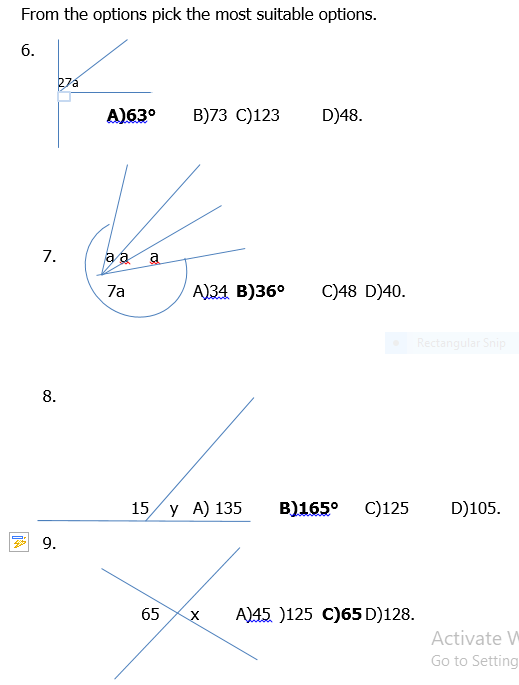### Mathematics JSS1 Third Term Final Assessment

ASSESSMENT.

From the options below select the suitable option as an answer.

1. An angle that is less than 90° is called A) Reflex B) Acute C) Obtuse D) Right-angled.

2. An angle that is greater than 180° but less than 360° is A)Obtuse B) Reflex C) Acute D) isosceles.

3. An angle that is equal to 90° is A) Right-angled B) Obtuse C) Reflex D) straight.

4. An angle that is half a turn is A) straight B) Acute C)Obtuse D) Reflex.

5. An angle that is greater than 90° but less than 180° is A)Acute B)Reflex C)Obtuse D)Straight.Use the data presented in the table to answer question 1-2 and select the correct answer from the options.

View More